For the network shown in the figure the value of the current i is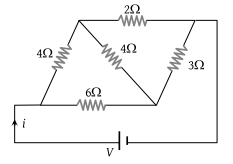(1) $\frac{9V}{35}$

(2) $\frac{5V}{18}$

(3) $\frac{5V}{9}$

(4) $\frac{18V}{5}$

Concept Questions :-

Combination of resistors
High Yielding Test Series + Question Bank - NEET 2020

Difficulty Level:

When a wire of uniform cross-section a, length l and resistance R is bent into a complete circle, the resistance between any two of diametrically opposite points will be :

(1) $\frac{R}{4}$

(2) $\frac{R}{8}$

(3) 4R

(4) $\frac{R}{2}$

Concept Questions :-

Combination of resistors
High Yielding Test Series + Question Bank - NEET 2020

Difficulty Level:

In the circuit given E = 6.0 V, R1 = 100 ohms, R2 = R3 = 50 ohms, R4 = 75 ohms. The equivalent resistance of the circuit, in ohms, is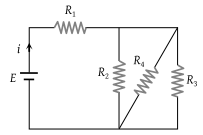(1) 11.875

(2) 26.31

(3) 118.75

(4) None of these

Concept Questions :-

Combination of resistors
High Yielding Test Series + Question Bank - NEET 2020

Difficulty Level:

By using only two resistance coils-singly, in series, or in parallel one should be able to obtain resistances of 3, 4, 12, and 16 ohms. The separate resistances of the coil are :

(1) 3 and 4

(2) 4 and 12

(3) 12 and 16

(4) 16 and 3

Concept Questions :-

Combination of resistors
High Yielding Test Series + Question Bank - NEET 2020

Difficulty Level:

In the given circuit, the voltmeter records 5 volts. The resistance of the voltmeter in ohms is :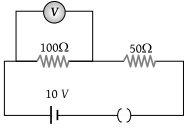(1) 200

(2) 100

(3) 10

(4) 50

Concept Questions :-

Kirchoff's voltage law
High Yielding Test Series + Question Bank - NEET 2020

Difficulty Level:

In the adjoining circuit, the battery E1 has an e.m.f. of 12 volts and zero internal resistance while the battery E has an e.m.f. of 2 volts. If the galvanometer G reads zero, then the value of the resistance X in ohm is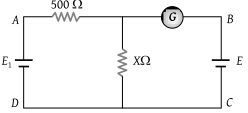(1) 10

(2) 100

(3) 500

(4) 200

Concept Questions :-

Grouping of cells
High Yielding Test Series + Question Bank - NEET 2020

Difficulty Level:

The magnitude and direction of the current in the circuit shown will be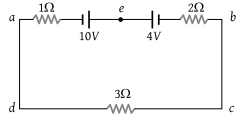(1) $\frac{7}{3}$A from a to b through e

(2) $\frac{7}{3}$A from b to a through e

(3) 1A from b to a through e

(4) 1A from a to b through e

Concept Questions :-

Grouping of cells
High Yielding Test Series + Question Bank - NEET 2020

Difficulty Level:

The e.m.f. of a cell is E volts and internal resistance is r ohm. The resistance in external circuit is also r ohm. The p.d. across the cell will be

(1) E/2

(2) 2E

(3) 4E

(4) E/4

Concept Questions :-

Emf and terminal voltage
High Yielding Test Series + Question Bank - NEET 2020

Difficulty Level:

Kirchhoff's first law i.e. $\Sigma i=0$ at a junction is based on the law of conservation of :

(1) Charge

(2) Energy

(3) Momentum

(4) Angular momentum

Concept Questions :-

Kirchoff's current law
High Yielding Test Series + Question Bank - NEET 2020

Difficulty Level:

The figure below shows currents in a part of electric circuit. The current i is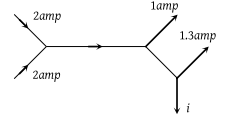(1) 1.7 amp

(2) 3.7 amp

(3) 1.3 amp

(4) 1 amp

Concept Questions :-

Kirchoff's current law